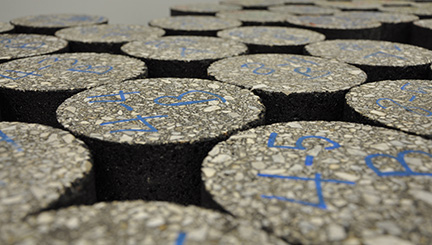# A better way to measure RAP and core Gmm

Find theoretical specific gravity of RAP and cored material

By Mark D. Blow, P.E.

Crushing, milling and saw cutting can result in uncoated faces of recycled asphalt pavement (RAP) particles and core samples. Those uncoated faces often complicate determining the maximum theoretical specific gravity (Gmm).

Uncoated faces allow water to be absorbed into the samples and require the technician to institute the dry-back procedure contained in AASHTO T209/ASTM D2041. The dry-back procedure is time-consuming and introduces unnecessary error into the process. There is a better way.

In the asphalt world, all specific gravities boil down to a simple “Mass/Volume” equation. This can be easily observed when studying the AASHTO T209, “Mass Determination in Air” equation:

Theoretical Maximum Specific Gravity = A / A+D–E

Where :
A = mass of the oven-dry sample in air, in grams (g);
D = mass of the container filled with water at 25°C (77°F), g; and

E = mass of the container filled with the sample and water  at 25°C (77°F), g.

Upon observation, it is evident that the numerator of the above equation is a mass, but is the denominator actually a volume? The answer is “yes.”

If you add the mass of the oven-dry sample in air to the mass of the container filled with water and subtract the mass of the container filled with the sample and water, what are you missing? The mass of the water that was in the container but has been displaced by the sample of mix. Hence the denominator is the mass of the displaced water which for all practical purposes equals the volume of the mix in the container since the density of water is approximately 1.0 gram per cubic centimeter at 25°C (77°F).

Why does this matter? There is a simple solution that will prevent intrusion of water into your sample without the use of a film or plastic baggie — just add more asphalt to it!

Simply preheat the mix to be tested to 60 to 120°C as you would during a normal mix design, place it in the mixing bowl and add one to two percent binder to thoroughly coat the mixture and let it cool. If a large amount of uncoated particles are present, a 2-hour curing time should be more than sufficient for the purposes of this test. When Gmm testing is completed, simply subtract the added asphalt mass and volume from the original procedure using the following equation:

Theoretical Maximum Specific Gravity = A–J / (A+D) – (E+K)

Where:

A = mass of the oven-dry sample in air, g;
D = mass of the container filled with water at 25°C (77°F), g;
E = mass of the container filled with the sample and water at 25°C (77°F), g;
J = mass of the added asphalt binder in air, g;
K = volume of the added asphalt binder, cc or ml = J/Gb
Where Gb = the specific gravity of the binder added.

Due to the variability of aggregate materials and their respective absorption rates, technicians may wish to experiment with the range of added asphalt. The desired goal is to thoroughly coat the sample, but not excessively, to where drain down can occur. The newly coated sample will resist water intrusion, minimize the presence of fugitive dust (cloudy water) and provide reliable, accurate results.

Have confidence in your Gmm results. Try the Asphalt Institute Gmm RAP Method today.